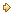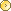Visitors Online: 77 | Monday 27th May 2019

CBSE Guess > Papers > Question Papers > Class XII > 2004 > Economics > Outside Delhi Set-I

ECONOMICS (Set I—Outside Delhi)

SECTION – A

Q. 1. Answer the following questions: 1x4
(i) What is meant by price elasticity of demand?
(ii) In which market form are the products homogeneous?
(iii) Define marginal revenue.
(iv) State the law of supply.

Q. 2. Mention any three factors that affect the price elasticity of demand of a commodity. 3

Q. 3. Distinguish between ‘change in demand’ and ‘change in quantity demanded’ of a commodity. 3

Q. 4. List any three determinants of supply of a commodity. 3

Q. 5. State any three main features of monopolistic competition. Describe any one. 3

Q. 6. The quantity supplied of a commodity at a price of Rs. 8 per unit is 400 units. Its price elasticity of supply is 2. Calculate the price at which its quantity supplied will be 600 units. 4

Q. 7. Complete the following table: 4

 Output (unit) Price (Rs.) Total Revenue (Rs.) Margial Revenue (Rs.) 1 2 3 4 7 6 4 2 — — — — — — — —

Q. 8. Explain the relationship between marginal cost and average cost with the help of a cost schedule. 4
Or
Distinguish between fixed costs and variable costs. Give two examples of each.

Q. 9. What are the three central problems of an economy? Why do they arise? 4

Q. 10. Explain with the help of diagrams the effect of the following changes on the demand of a commodity: 6
(i) A fall in the price of complementary good
(ii) A rise in the income of its buyer

Q. 11. Explain the law of variable proportions with the help of total product and marginal product curves. 6

Q. 12. If at a given price of a commodity there is, excess supply, how will the equilibrium price be reached? Explain with the help of a diagram. 6
Or
Explain the effect of a leftward shift of demand curve of a commodity on its equilibrium price and quantity, with the help of a diagram.

SECTION - B

Q. 13. Answer the following questions: 1x4
(i) What is macro-economics?
(ii) Give an example of a micro-economic study.
(iii) What is meant by fiscal deficit?
(iv) When is there a deficit in the balance of trade?

Q. 14. What is meant by revenue deficit? What are the implications of this deficit? 3

Q. 15. Calculate Net National Disposable Income from the following data: 3

 Rs. (Crores) (i) Gross national product at factor cost (ii) Net current transfers from rest of the world (iii) Net indirect tax (iv) Consumption of fixed capital (v) Net factor income from abroad 800 50 70 60 (-) 10

Q. 16. Give the meaning of marginal propensity to save and aver age propensity to save. Can the value of average propensity to save benegative? If yes, when?3

Q. 17. In an economy, the marginal propensity to consume is 0.75. Investment is increased by Rs. 200 crores. Calculate the total increase in income and consumption expenditures. 3

Q. 18. How does a central bank control the availability of credit by open market operations? Explain. 4

Q. 19. Explain briefly any two objectives of a government budget. 4

Q. 20. State the four functions of money. Describe any one. 4

Q. 21. Distinguish between current account and capital account of balance of payments account. Mention any two transactions of capital account. 4
Or
How is the foreign exchange market rate determined? Explain with the help of a diagram.

Q. 22. From the following data calculate National Income by (i) income method and (ii) expenditure method: 3, 3

 Rs. (Crores) (i) Compensation of employees (ii) Net factor income from abroad (iii) Net indirect tax (iv) Profits (v) Private final consumption expenditure (vi) Net domestic capital formation (vii) Consumption of fixed capital (viii) Rent (ix) Interest (x) Mixed income of self-employed (xi) Net exports (xii) Government final consumption expenditure 1,200 (-) 20 120 800 2,000 770 130 400 620 700 (-) 30 1, 100

Q. 23. Will the following be included in domestic factor Income of India? Give reasons for your answer. 6
(i) Profits earned by a foreign bank from its branches Ii India.
(ii) Scholarships given by Government of India.
(iii) Profits earned by a resident of India from his company In Singapore.
(iv) Salaries received by Indians working In American Embassy in India.

Q. 24. Explain the concept of under-employment equilibrium with the help of a diagram. Show on the same diagram the additional in vestment expenditure required to reach full employment equilibrium. 6
Or
Explain the equilibrium level of income with the help of Consumption + Investment (C + I) curve If planned saving is greater than planned Investment, what adjustments will bring about equality between the two?

 Economics 2004 Question Papers Class XII Delhi Outside Delhi Compartment Delhi Compartment Outside DelhiSet ISet ISet ISet ISet IISet IISet IISet IISet IIISet III

 CBSE 2004 Question Papers Class XIIEnglishSociologyFunctional EnglishPsychologyMathematicsPhilosopyPhysicsComputer ScienceChemistryEntrepreneurshipBiologyInformatics PracticesGeographyMultimedia & Web TechnologyEconomicsBiotechnologyBusiness StudiesPhysical EducationAccountancyFine ArtsPolitical ScienceHistoryAgriculture